Courses

# Test: Alligation Or Mixture- 3

## 15 Questions MCQ Test Quantitative Aptitude (Quant) | Test: Alligation Or Mixture- 3

Description
This mock test of Test: Alligation Or Mixture- 3 for Quant helps you for every Quant entrance exam. This contains 15 Multiple Choice Questions for Quant Test: Alligation Or Mixture- 3 (mcq) to study with solutions a complete question bank. The solved questions answers in this Test: Alligation Or Mixture- 3 quiz give you a good mix of easy questions and tough questions. Quant students definitely take this Test: Alligation Or Mixture- 3 exercise for a better result in the exam. You can find other Test: Alligation Or Mixture- 3 extra questions, long questions & short questions for Quant on EduRev as well by searching above.
QUESTION: 1

### Two types of oils having the rates of Rs. 4/kg and Rs. 5/kg respectively are mixed in order to produce a mixture having the rate of Rs. 4.60/kg. What should be the amount of the second type of oil if the amount of the first type of oil in the mixture is 40 kg?

Solution:

Mixing Rs. 4/kg and Rs. 5/kg to get Rs. 4.6 per kg we get that the ratio of mixing is 2:3. If the first oil is 40 kg, the second would be 60 kg

QUESTION: 2

### A mixture of 125 gallons of wine and water contains 20% water. How much water must be added to the mixture in order to increase the percentage of water to 25% of the new mixture?

Solution:

In 125 gallons we have 25 gallons water and 100 gallons wine. To increase the percentage of water to 25%, we need to reduce the percentage of wine to 75%. This means that 100 gallons of wine = 75% of the new mixture. Thus the total mixture = 133.33 gallons. Thus, we need to mx 133.33 - 125 = 8.33 gallons of water in order to make the water equivalent to 25% of the mixture.

QUESTION: 3

### 400 students took a mock exam in Delhi. 60% of the boys and 80% of the girls cleared the cut off in the examination. If the total percentage of students qualifying is 65%, how many girls appeared in the examination?

Solution:

The ratio of boys and girls appearing for the exam can be seen to be 3:1 using the following alligation figure.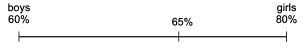This means that out of 400 students, there must have been 100 girls who appeared in the exam.

QUESTION: 4

The average salary per head of all employees of a company is Rs. 600. The average salary of 120 officers is Rs. 4000. If the average salary per head ofthe rest of the employees is Rs. 560, find the total number of workers in the company.

Solution: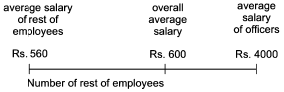From the figure it is clear that the ratio of the number of officers to the number of other employees would be 40:3400. Since there are 120 officers, there would be 3400 x 3 = 10200 workers in the company. Thus the total number of employees would be 10200 + 120 = 10320.

QUESTION: 5

A cistern contains 50 litres of water. 5 litres of water is taken out of it and replaced by wine. The process is repeated again. Find the proportion of wine and water in the resulting mixture.

Solution:

Amount of water left = 50 x 9/10 x 9/10 = 40.5 liters. Hence, wine = 9.5 liters. Ratio of wine and water = 19:81. Option (c) is correct.

QUESTION: 6

A vessel is full of refined oil. 1/4 of the refined oil is taken out and the vessel is filled with mustard oil. If the process is repeated 4 times and 10 litres of refined oil is finally left in the vessel, what is the capacity of the vessel?

Solution: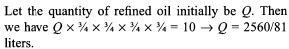QUESTION: 7

A thief steals four gallons of liquid soap kept in a train compartment’s bathroom from a container that is full of liquid soap. He then fills it with water to avoid detection. Unable to resist the temptation he steals 4 gallons of the mixture again, and fills it with water. When the liquid soap is checked at a station it is found that the ratio of the liquid soap now left in the container to that of the water in it is 36 : 13. What was the initial amount of the liquid soap in the container if it is known that the liquid soap is neither used nor augmented by anybody else during the entire period?

Solution:

It can be seen from the ratio 36:13 that the proportion of liquid soap to water is 36/49 after two mixings. This means that 6/7th of the liquid soap must have been allowed to remain in the container and hence l/7th of the conatiner’s original liquid soap would have been drawn out by the thief. Since he takes out 4 gallons every time, there must have been 28 gallons in the container. ( as 4 should be l/7th of 28)

QUESTION: 8

A sum of Rs. 36.90 is made up of 90 coins that are either 20 paise coins or 50 paise coins. Find out how many 20 paise coins are there in the total amount,

Solution:

The average value of a coin is 41 paise and there are only 20 paise and 50 paise coins in the sum. Hence, the ratio of the number of 20 paise coins to 50 paise coins would be 9:21 = 3:7. Since there are a total of 90 coins, the number of 20 paise coins would be 3 x 90/10 = 27 coins.

QUESTION: 9

A mixture of 70 litres of alcohol and water contains 10% of water. How much water must be added to the above mixture to make the water 12.5% of the resulting mixture?

Solution:

Solve using options. Initially there are 7 liters of water in 70 liters of the mixture. By mixing 2 liters of water we will have 9 liters of water in 72 liters of the mixture - which is exactly 12.5%.

QUESTION: 10

A merchant purchased two qualities of pulses at the rate of Rs. 200 per quintal and Rs. 260 per quintal. In 52 quintals of the second quality, how much pulse of the first quality should be mixed so that by selling the resulting mixture at Rs. 300 per quintal, he gains a profit of 25%?

Solution:

By selling at 300 if we need to get a profit of 25% it means that the cost price would be 300/1.25 = 240.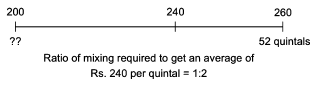Thus, in 52 quintals of the second we need to mix 26 quintals of the first.

QUESTION: 11

There are two mixtures of honey and water, the quantity of honey in them being 25% and 75% of the mixture. If 2 gallons of the first are mixed with three gallons of the second, what will be the ratio of honey to water in the new mixture?

Solution:

The percentage of honey in the new mixture would be: (2 x 25 + 3 x 15)15 = 275/5 =55%. The ratio of honey to water in the new mixture would be 55:45 = 11:9.

QUESTION: 12

Two containers of equal capacity are full of a mixture of oil and water. In the first, the ratio of oil to water is 4 : 7 and in the second it is 7 : 11. Now both the mixtures are mixed in a bigger container. What is the resulting ratio of oil to water?

Solution:

Assume the capacity of the two containers is 198 liters each. When we mix 198 liters of the first and 198 liters of the second the amount of oil would be: 198 x 4/11 + 198 x 7/18 = 72 + 77 = 149 liters. Consequently the amount of water would be 396 - 149 = 247 liters. Option (a) is correct.

QUESTION: 13

The price of a pen and a pencil is Rs. 35. The pen was sold at a 20% profit and the pencil at a 10% loss. If in the transaction a man gains Rs. 4, how much is cost price of the pen?

Solution:

Solve using options as that would be the best way to tackle this question. Option (b) fits the situation perfectly as if we take the price of the pen as Rs. 25, the cost of the pencil would be Rs. 10. The profit in selling the pen would be Rs.5 while the loss in selling the pencil would be Rs. 1. The total profit would be Rs. 4 as stipulated by the problem.

QUESTION: 14

A vessel is full of a mixture of kerosene and petrol in which there is 18% kerosene. Eight litres are drawn off and then the vessel is filled with petrol. If the kerosene is now 15%, how much does the vessel hold?

Solution:

The following visualization would help: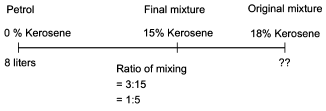From the figure we can see that the original mixture would be 40 liters and the petrol being mixed is 8 liters. Thus, the vessel capacity is 48 liters.

QUESTION: 15

In the Singapore zoo, there are deers and there are ducks. If the heads are counted, there are 180, while the legs are 448. What will be the number of deers in the zoo?

Solution:

If all the animals were ducks we would have 180 heads and 360 legs. If we reduce the number of ducks by 1 to 179 and increase the number of deers by 1 to 1, we would get an incremental 2 legs.
Since, the number of legs we need to increment is 88 (448 - 360 = 88), we need to have 44 deers and 136 ducks.